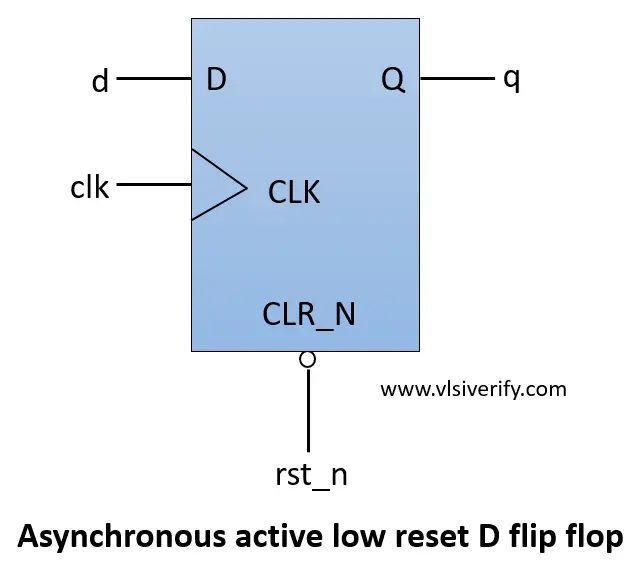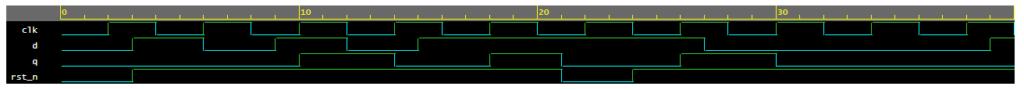Tutorials

The D flip flop is a basic sequential element that has data input ‘d’ being driven to output ‘q’ as per clock edge. Also, the D flip-flop held the output value till the next clock cycle. Hence, it is called an edge-triggered memory element that stores a single bit.

The below D flip flop is positive edge-triggered and has asynchronous active low reset.

As soon as reset is triggered, the output gets reset## D Flip Flop with Asynchronous Reset Verilog Code

``````module D_flipflop (
input clk, rst_n,
input d,
output reg q
);

always@(posedge clk or negedge rst_n) begin
if(!rst_n) q <= 0;
else       q <= d;
end

endmodule``````

## Testbench Code

``````module tb;
reg clk, rst_n;
reg d;
wire q;

D_flipflop dff(clk, rst_n, d, q);

always #2 clk = ~clk;
initial begin
clk = 0; rst_n = 0;
d = 0;

#3 rst_n = 1;

repeat(6) begin
d = \$urandom_range(0, 1);
#3;
end
rst_n = 0; #3;
rst_n = 1;
repeat(6) begin
d = \$urandom_range(0, 1);
#3;
end
\$finish;
end

initial begin
\$dumpfile("dump.vcd");
\$dumpvars(1);
end
endmodule``````

## WaveformVerilog Codes